# Spatial-Temporal Correlation-Based LSTM Algorithm and Its Application in PM2.5 Prediction

Spatial-Temporal Correlation-Based LSTM Algorithm and Its Application in PM2.5 Prediction
Department of Mathematics and Computer Science, Hebei Normal University for Nationalities, Chengde 067000, China

Corresponding Author Email:
zhaoyanming008@163.com
Page:
29-38
|
DOI:
https://doi.org/10.18280/ria.340104
10 September 2019
|
Accepted:
1 December 2019
|
Published:
29 February 2020
| Citation

OPEN ACCESS

Abstract:

In existing researches, the algorithms for simulating and predicting the evolution process of air pollutant particle concentration have neither explored the spatial correlation of particle concentration in depth, nor achieved the fusion of the time dependence and the spatial correlation of the particle concentration. To this end, this paper proposes the long-short term memory network (LSTM) algorithm based on spatiotemporal fusion. First, the spatial correlation, the relevant factors and the calculation methods are proposed; then, the local spatial correlation factors are combined with the forget gate and the remember gate of the LSTM algorithm to construct a LSTM algorithm based on local spatial information and spatial-temporal correlation, namely the LTS-LSTM; after that, the learning result of LTS-LSTM is combined with the global spatial correlation factors to construct a LSTM algorithm based on global spatial information and spatial-temporal correlation, namely the GTS-LSTM; at last, the proposed algorithm is adopted to simulate the global and local air pollution particle concentration evolution process, and predict the particle concentration. On the global and local observation dataset, the proposed algorithm is compared with the regression algorithm, support vector machine (SVM), fuzzy neural network (FNN), LSTM neural network, GC-LSTM neural network, and DL-LSTM neural network. The comparison results show that: in terms of air particle concentration prediction, the performance of the proposed algorithm outperforms other traditional prediction algorithms, and its performance is close to the deep LSTM algorithms.

Keywords:

long-short term memory (LSTM) network, air pollutant concentration prediction, recurrent neural network (RNN), spatial-temporal correlation, PM2.5 concentration

1. Introduction

Air pollution is closely related to human health. In 2013, the research of Zheng et al.  showed that real-time prediction of the concentration of air pollutant particles is of great significance for controlling air pollution and reducing health problems caused by air pollution. The research of Di et al.  in 2017 and the research of Hung et al.  in 2018 respectively pointed out that: for the particles, the smaller the volume, the stronger the water solubility, the stronger the penetrability into the respiratory system, the higher the adsorption rate, and the greater the impact on human health. Therefore, the evolution process of PM2.5 particle concentration and the prediction algorithms have become hot topics in current studies.

At present, the prediction algorithms for the evolution process of the concentration of air pollutant particles mainly include two categories: the process model algorithms and the statistical algorithms. Wherein the process model algorithms [4-7] construct models based on the prior knowledge of meteorological theories and the atmospheric physicochemical reaction processes to achieve modeling, simulation and concentration prediction of the evolution process of the concentration of air pollutant particles. In terms of research areas and research methods, such algorithms studied the spatial-temporal distribution characteristics and evolution process of the concentration of air pollutant particles and achieved good research results. However, the algorithms proposed based on prior knowledge and atmospheric physicochemical process is a non-linear air quality prediction method constrained by multi-dimensional conditions, and these algorithms are not universal.

The statistical algorithms can overcome the shortcomings of the process model algorithms, such algorithms can be divided into non-neural network algorithms and neural network algorithms. Wherein the non-neural network algorithms include: multiple linear regression (MLR) , support vector regression (SVR) , wavelet-ARMA/ARIMA algorithm , etc. The statistical algorithms can overcome the shortcomings of the process model algorithms via regression, and achieved good simulation and prediction results in research and application fields.

The experiment conducted by Yoon et al.  in 2011 showed that artificial neural networks (ANN) have good application value in the simulation and prediction of the evolution process of the concentration of atmospheric pollutant particles. In the past ten years, various ANN algorithms have been designed at home and abroad to predict air pollutant concentration. ANN algorithms include common neural network algorithms and special neural network algorithms. Common neural network algorithms include: air quality prediction algorithm based on radial basis function neural network (RBFNN) , air quality prediction algorithm based on multi-layer perceptron (MLP) , time delay neural network (TDNN) , Elman neural network , and air prediction algorithm based on fuzzy neural network (FNN) . Algorithms of this type generally apply different neural network algorithms to the simulation of the evolution of atmospheric pollutant particles and the prediction of particle concentration, and can achieve good results. However, these algorithms have ignored that the research object is the feature of time series, and they ought to start from the perspective of time series to learn the time-dependent feature of the process.

Therefore, in 2011, Feng et al.  applied the recurrent neural network (RNN) based on time-dependent feature learning to air quality prediction and achieved good results. In 2015, Ma et al.  pointed out that RNN can effectively extract the time-dependent feature of time series, and ensure the ability to learn time series. However, the problems of gradient vanishing or gradient exploding have restricted the long-term time-dependent feature of RNN in learning time series. Therefore, in 1997, Hochreiter and Schmidhube developed a LSTM neural network (LSTM NN) to solve the gradient problems of traditional RNNs and realize the long-term time dependence learning of time series.

In recent years, LSTM algorithms have achieved good research results in the field of simulation and prediction of the evolution process of air pollutant particle concentration, and the representative algorithms include: LSTM method and evaluation algorithm , ensemble-LSTM algorithm , CNN-LSTM algorithm , LSTM-FC algorithm ; LSTM algorithms based on air pollutant particle concentration characteristics: GC-LSTM algorithm , spatiotemporal convolutional LSTM algorithm ; LSTM algorithm based on deep learning: DL-LSTM algorithm , Multi-output DL-LSTM algorithm ; Deep DL-LSTM algorithm . Algorithms of this type took the LSTM algorithm as the core, starting from the structural characteristics of the research object, they improved the LSTM algorithm to effectively simulate the evolution process of the concentration of atmospheric pollutant particles and improve the prediction performance of the algorithm, moreover, based on data analysis, they introduced CNN, FC, GC, DL, and other algorithms to optimize the input data and preliminarily explored the spatial correlation of the evolution process of particle concentration.

In summary, the LSTM-based atmospheric particle concentration evolution process simulation and concentration prediction algorithms have achieved good results, but still, they have the following shortcomings: 1) These algorithms have ignored the spatial correlations of the observation space, including global and local spatial correlations; 2) These algorithms have not explored the spatial correlation of the evolution process of the concentration of atmospheric particles; 3) These algorithms have not effectively integrated spatial correlation into the logic gate of the LSTM algorithms to achieve LSTM improvement in time dependence and spatial correlation or applied them to simulate and predict the evolution process of the concentration of atmospheric pollutant particles.

To solve these problems, this paper proposes a LSTM algorithm based on spatial-temporal correlations and applies it to PM2.5 concentration prediction. The main innovations in this paper include: 1) It proposes the global and local spatial correlations and their calculation methods to achieve the universality of the algorithm. 2) It proposes to combine the local spatial information correlation factors with the forget gate and remember gate of LSTM algorithm to construct the LTS-LSTM algorithm based on local spatial information, so that the LSTM algorithm has the ability to learn local spatial information features. 3) With the learning result of LTS-LSTM algorithm as the input, this study combines with the global spatial correlation to construct the GTS-LSTM algorithm based on global spatial information, then from the global perspective of geographic information, it stimulates the evolution process of the concentration of air pollutant particles and predicts the particle concentration.

2. The LSTM Algorithm Based on Spatial-Temporal Correlations

The evolution process of the particle concentration of atmospheric pollutant PM2.5 has high time dependence and spatial correlation. Therefore, constructing a LSTM NN with temporal and spatial memory functions enables us to better simulate the evolution process of PM2.5 and accurately predict the PM2.5 particle concentration.

2.1 PM2.5 particle concentration has spatial correlation

Feng et al.  preliminary studied the geographical correlation of the evolution process of the concentration of atmospheric pollutant particles, and pointed out that the geographical correlation factors include wind strength, wind direction and geographical location. However, these literatures have ignored the relationship between geographic correlation and the scope of the research area. Therefore, according to the scope of the research area, this paper divides the research area into two types: global spatial area and local spatial area. In local area, geographical correlation factors include wind strength, wind direction, and geographic location; in global area, the geographical correlation is also affected by key spatial factors such as mountains and vegetation, etc. For different areas, the key factors that determine the geographical correlation are different as well. Therefore, the spatial correlation information diagram is drawn as follows:

## 1.pngFigure 1. Spatial correlation information

In Figure 1, pi and pj represent two adjacent air quality monitoring stations; Mountain_coefficient represents the mountain influence coefficient of the PM2.5 particle concentration between the two adjacent points pi and pj, and this coefficient is related to the span, height of the mountain, and the angle between the mountain and the connection line between pi and pj, it’s defined as Mountain_coefficient$=M_{-}$length$\times M_{-}$width$\times$ $M_{-} h i g h \times \cos \theta ;$ this coefficient has good slow-varying feature and wind power instantaneity, it is a long-term and dynamic changing process, the larger the area, the stronger the stability. Wind_coefficient represents the wind influence coefficient of the PM2.5 particle concentration between the two adjacent points pi and pj, this coefficient is related to the wind strength, wind direction, and the angle between the wind direction and the connection line between $p_{i}$ and $p_{j},$ it's defined as Wind_coefficient $=$ power_wind $\times \cos \varphi .$ Vegetation_coefficient represents the vegetation influence coefficient of the PM2.5 particle concentration between the two adjacent points pi and pj, this coefficient is related to the luxuriant degree of the vegetation between pi and pj, here we use the NDVI coefficient to represent Vegetation_coefficient; this coefficient can reflect the flourishing degree of  vegetation in this area, and it is less affected by other conditions. D(j,i) represents the distance between pj and pi, here we use the Euclidean distance of the latitude and longitude between the two points to represent it.

Formula (1) represents the geospatial information $\tau(i, j)$ of the PM2.5 particle concentration, this information indicates the correlation between the particle concentration at the neighbor observation point and the particle concentration at the current observation point.

$\tau(i, j)=$ Wind_cofficent $\times$ Mountain_cofficent $\times$ Vegetation_cofficent/D $(i, j)$         (1)

In global space, the real-time attributes of wind (strength and direction), geographical location, regional mountains, and regional vegetation are all key influencing factors and are all affected by time lag. In local area, the distance between observation points is relatively close. The key factors that determine the geospatial information correlation include the real-time attributes of the wind (strength and direction), and the geographical location. Therefore, Formula (1) is converted into.

$\tau(i, j)=$$\left\{\begin{array}{cc}W_{-} \text {wind } \times \cos \theta \times M_{-} \text {mountain} & \text {Globalarea} \\ \times \cos \varphi \times \operatorname{NDVI} / D(i, j) & \text {} \\ W_{-} \text {wind} \times \cos \theta / D(i, j) & \text {localarea}\end{array}\right.$           (2)

Formula (2) is taken as the calculation criterion of local spatial correlation to complete the local area spatial information correlation calculation and generate local geographic correlation vectors with real-time features. Therefore, the local spatial correlation coefficient matrix and the global spatial correlation coefficient matrix are:

$\pi=\left[\begin{array}{ccccc}\pi 11 & \pi 12 & \pi 13 & & \pi 1 n \\ \pi 21 & \pi 22 & \pi 23 & & \pi 2 n \\ \pi 31 & \pi 32 & \pi 33 & & \pi 3 n \\ & & & & \\ \pi n 1 & \pi n 2 & \pi n 3 & & \pi n n\end{array}\right]$

$\tau=\left[\begin{array}{ccccc}\tau 11 & \tau 12 & \tau 13 & & \tau 1 n \\ \tau 21 & \tau 22 & \tau 23 & & \tau 2 n \\ \tau 31 & \tau 32 & \tau 33 & & \tau 3 n \\ & & & & \\ \tau n 1 & \tau n 2 & \tau n 3 & & \tau n n\end{array}\right]$

2.2 LSTM algorithm

LSTM network is a special type RNN which consists of an input layer, an output layer, and a series of repeatedly connected hidden layers. It implements time-dependence learning in the form of threshold and has many variants. This paper adopts the LSTM algorithm with the following structure (Figure 2).

## 2.png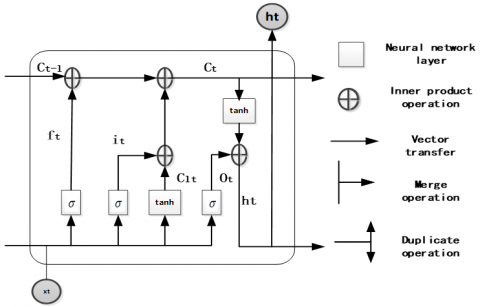Figure 2. LSTM neural network structure

Here, the input vector of the LSTM algorithm is denoted as $\mathrm{ℵ}=\left(x_{1}, x_{2}, x_{3}, \ldots x_{n}\right), x_{i} \in R^{T}, i=1,2,3, \ldots, n ;,$ and $\mathrm{n}$ represents the number of dimensions of the input vector. T represents the time lag of the time series. Y=(y1,y2,y3,...yn) represents the output series; then the learning process of the LSTM algorithm is described as:

$f_{t}=\sigma\left(W_{f} \cdot\left[h_{t-1}, \mathrm{x}_{t}\right]+b_{f}\right)$           (3)

$i_{t}=\sigma\left(W_{i} \cdot\left[h_{t}, x_{t}\right]+b_{i}\right)$             (4)

$C_{t}=f_{t} * C_{t-1}+i_{t} * C_{t}$                (5)

$o_{t}=\sigma\left(W_{o} \cdot\left[h_{t-1}, x_{t}\right]+b_{o}\right)$                         (6)

$h_{t}=o_{t} * \tanh \left(C_{t}\right)$       (7)

where, it, ot and ft respectively represent the input gate, output gate, and forget gate of the LSTM network; ct and ht respectively represent the activation vector of each neuron cell and memory module; w and b respectively represent the weight matrix and the bias vector; in addition, $\sigma(\cdot)$ represents the activation function, which is defined as the Formula (8); $\tanh (\cdot)$ represents hyperbolic tangent function tanh (), and it is defined as Formula (9).

$\sigma(x)=\frac{1}{1+e^{-x}}$         (8)

$\tanh (x)=\frac{e^{x}-e^{-x}}{e^{x}+e^{-x}}$                         (9)

This paper adopts the BPTT algorithm to train the LSTM network.

2.3 LSTM algorithm that fuses geospatial information

The PM2.5 particle concentration observation series is a long-term time-dependent time series. The LSTM algorithm can well learn the time-dependent features of the time series but it cannot learn the spatial correlation of PM2.5 particle concentration. Based on this, this paper proposes a spatial-temporal correlation-based LSTM algorithm that combines the time-dependence and spatial correlation of PM2.5 particle concentration from the micro-units of the LSTM, enabling LSTM to fuse the learning of the time-dependence and the spatial correlation. Combining with the spatial classification of the particle concentration, here we propose the two-step simulation and prediction algorithm.

The first step: the LTS-LSTM algorithm that fuses local spatial correlation information. According to the spatial information logic switch (K=1), achieve the learning of the spatial correlation information of the local area. The functional structure of the LTS-LSTM algorithm is Figure 3:

## 3.png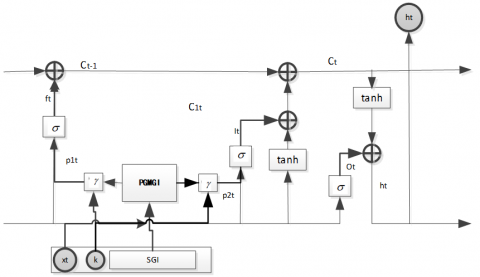Figure 3. Functional structure of the LTS-LSTM algorithm (PGMGI is the parameter generation module of the geographic information; SGI is the sequence of the geographic information)

Spatial correlation can accelerate or decelerate the evolution process of the concentration of PM2.5 particles, $\tau(i, j)>0$ indicates that the neighbor observation point j promotes the positive increase of PM2.5 particle concentration at observation point i, which is reflected in the increase in remembering and the decrease in forgetting, $P_{1 t}=1-\tau(i, j), P_{2 t}=\tau(i, j)$; conversely, it indicates that the neighbor observation point j promotes the positive decrease of PM2.5 particle concentration at observation point i, which is reflected in the decrease in remembering and the increase in forgetting, $P_{1 t}=1-|\tau(i, j)|, P_{2 t}=|\tau(i, j)|$.

Therefore, improve the Formulas (3) and (4) that respectively represent remembering and forgetting, and the improvement results are: $f_{t}=\sigma\left(W_{f} \cdot\left[h_{t-1}, \mathbf{x}_{t}, p_{1 t}\right]+b_{f}\right)$ and $i_{t}=\sigma\left(W_{i} \cdot\left[h_{t}, x_{t}, p_{2 t}\right]+b_{i}\right) .$ Introduce the spatial correlation information p1t and p2t into the calculation of remember gate and forget gate, combining the calculations of the spatial information correlation and time-dependence, and achieve the fused learning of local spatial correlation information and time-dependence information, wherein $P_{k t}=\tau(i, j)$.

The second step: GTS-LSTM algorithm that fuses global spatial correlation information. According to the geographic information logic switch (K=2), the global geographic information is integrated with the micro-output of the LSTM network to achieve the learning of the geographic information correlation of the global area (Figure).

## 4.png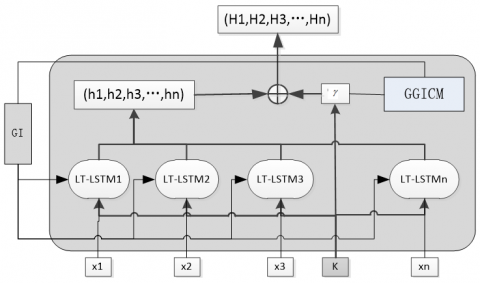Figure 4. GTS-LSTM algorithm function diagram (GI is the global geographic information; GGICM is the calculation module of global geographic information)

In global space, the distance between observation stations is relatively long, there might be mountains between the stations, and the influence of the mountains and vegetation on the evolution process of the concentration of PM2.5 particles is quite obvious, so it must be taken into account in the calculation; in the global area, according to the global spatial correlation coefficient matrix, the spatial correlation between each station is calculated. Therefore, the product of the LTS-LSTM output and the global spatial correlation coefficient matrix is calculated to realize the fused learning of global spatial correlation and time dependence. The result is: $H \times \pi(i)$.

After the two steps of the learning of spatial correlation and time-dependence, the algorithm has realized the fused learning of global spatial correlation and time dependence, and solved the problem that the LSTM network can only realize time-dependence learning, not spatial correlation learning. The proposed algorithm achieved the fused learning of global and local geographic information correlation and time-dependence, and explored the problem of how to solve spatial information in time series.

2.4 Evaluation methods for the prediction algorithms

This paper adopts three evaluation indicators to achieve the evaluation of the performance of the algorithms, namely the root means square error (RMSE), the mean absolute error (MAE), and the mean absolute percentage error (MAPE). The indicators can be expressed as follows:

$R M S E=\sqrt{\frac{1}{n} \sum_{i=1}^{N}\left(y_{i}-y_{i}^{*}\right)^{2}}$                         (10)

$M A E=\frac{1}{n} \sum_{i=1}^{n}\left|y_{i}-y_{i}^{*}\right|$                         (11)

$M A P E=\frac{1}{n} \sum_{i=1}^{n} \frac{\left|y_{i}-y_{i}^{*}\right|^{2}}{y_{i}^{*}}$                         (12)

where, $\mathcal{Y}_{i}^{*}$ is the observed air pollutant concentration, $y_{i}$ is the predicted air pollutant concentration, and n is the number of test samples.

3. Validation Dataset and Experimental Results

3.1 Algorithm research area and corresponding validation dataset

This paper chooses global dataset and local dataset to study the performance of the proposed algorithm. The global dataset: the Beijing-Tianjin-Hebei region, China, this region contains the main factors affecting the formation of PM2.5 in developing countries, so it has good representativeness. The data in the dataset was the 24-hour average PM2.5 particle concentration data collected by the National Meteorological Administration in the past seven years. The local area dataset: 12 air quality monitoring stations in Beijing, China, the dataset includes 24-hour PM2.5 particle concentration data. The global area and the local area datasets were divided into a test set and a training set with a ratio of 20:80 (Figure 5).

## 5.png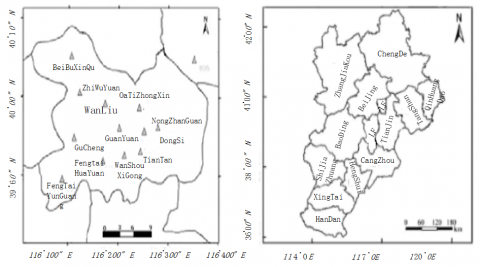a) Distribution map of local air quality monitoring stations in Beijing b) Distribution map of global air quality monitoring stations in Beijing-Tianjin-Hebei region

Figure 5. Global and local research areas

Note: Global area includes: north new district, Fengtai Yungang, National Agricultural Exhibition Center, Chengde, Langfang, Baoding, Shijiazhuang, Handan, Dongli, Jinnan, Development Zone and Wuqing District; Tianjin Economic-Technological Development Area; Local area includes: north new district, Botanical Garden, Wanliu, Olympic Sports Center, National Agricultural Exhibition Center, Dongsi, Guanyuan, Gucheng, Temple of Heaven, Wanshou West Palace, Fengtai Garden and Fengtai Yungang

3.2 Spatial correlation of PM2.5 particle concentration

Pearson correlation coefficient can accurately describe the correlation of the data. In this paper, this coefficient is used to research the spatial correlation of PM2.5 pollutant particle concentration, calculate the spatial correlation coefficient of particle concentration at different observation stations in the global area (Beijing-Tianjin-Hebei) and local area (Beijing), and plot the distribution maps of spatial correlation (Figure 6).

The experimental results show that, in the 1-hour time-lag area, the Pearson correlation coefficient of PM2.5 concentration at 12 air monitoring stations in Beijing was higher than 0.8, and the correlation coefficient of adjacent stations was higher than 0.91. Therefore, the PM2.5 concentration of the 12 observation stations had a strong spatial correlation, and the correlation of the adjacent stations was higher than that of the distant stations. In the 36-hour time-lag area, the Pearson correlation coefficient of PM2.5 concentration at 12 air monitoring stations in Beijing was higher than 7.7, and the correlation coefficient of the adjacent stations was higher than 0.89. Therefore, the PM2.5 concentration of the 12 observation stations had a strong spatial correlation, and the correlation of the adjacent stations was higher than that of the distant stations. In summary, in the appropriate time-lag areas, there’s strong correlation between adjacent global and local spaces, and the local spatial correlation was higher than the global spatial correlation.

## 6a.png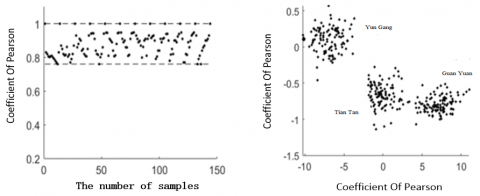a) Local area Pearson coefficient distribution map with 1-hour time-lag coefficient

## 6b.png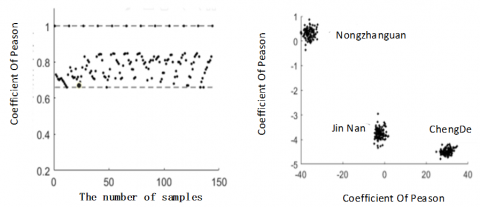b) Global area Pearson coefficient distribution map with 36-hour time-lag coefficient

Figure 6. Pearson correlation coefficient distribution of PM2.5 particle concentration

3.3 Long-term time-dependence of PM2.5 particle concentration

This paper uses the autocorrelation coefficient method to analyze the time dependence of the time series, and calculates the autocorrelation coefficients of the PM2.5 concentration samples from 12 air quality monitoring stations in the global area, these samples covered all four seasons in a year, for each season, 75 samples were collected; the autocorrelation coefficient of the PM2.5 concentration of the samples within 36 hours in the local area was calculated and a diagram of the correlation coefficient was plotted as follows (Figure 7).

## 7.pnga) Local spatial time dependence                     b) Global spatial time dependence

Figure 7. Relationship between time-lag and PM2.5 particle concentration autocorrelation coefficient in local and global spaces

The experimental results show that, in local and global spaces, there’s a long-term time dependence in the particle concentration between observation stations, and the time-lag relationship was quite clear. Compared with the long-term time dependence of local area, the time-lag of the long-term time dependence of global area is much longer.

3.4 Time-lag of PM2.5 particle concentration and algorithm performance

Time-lag can restrict the learning performance of time series algorithms. The evolution process of the concentration of PM2.5 particles is affected by time lag. Therefore, this paper took RMSE, MAE and MAPE as the three indicators to evaluate the time lag of the algorithms for the local and global areas. The evaluation results are (Table 1).

The experimental results show that the performance of the proposed algorithms is related to the time lag; as the time lag increased, the three error evaluation indicators of the algorithms were reduced, and the prediction performance of the algorithms was enhanced. We took both the performance of the algorithms and the time-space complexity into consideration, when the global area time lag is 12 hours and the local area time lag is 6 hours, the LTS-LSTM and GLS-LSTM algorithms performed best. Compared with the local area, in the global area, the key factors affecting the PM2.5 particle concentration are more complicated. The measuring point distance, the scale of the mountain and the vegetation between the measuring points all have a significant influence on the prediction algorithms, and these factors directly cause the global time lag to be longer than the local time lag.

Table 1. Performance of time-lag algorithms

 Types Local Area Global Area Evaluation index RMSE MAE MAPE Evaluation index RMSE MAE MAPE Lag Time 2 14.325 6.37 12.20 Lag Time 4 18.75 8.83 15.53 4 13.014 5.32 11.17 8 16.43 7.85 14.41 6 11.22 5.05 9.82 12 15.44 7.51 10.54 8 11.13 4.97 8.94 24 15.31 7.43 10.21 12 10.92 4.82 8.67 36 15.27 7.68 9.97

3.5 LSTM network structure and algorithm performance

The structure of the LSTM network, especially the number of nodes, has an important impact on the learning of long-term time dependence and geographic information correlation features. Therefore, for the 1-hour lag-time local space and the 36-hour lag-time global space, this study set three criteria to evaluate the influence of different network node numbers on the performance of the algorithms, the evaluation results are (Table 2):

Table 2. Influence of TS-LSTM neural network structure on algorithm performance

 Local Area Global Area Evaluation index RMSE MAE MAPE Evaluation index RMSE MAE MAPE Number of nodes 400 12.01 5.21 8.62 Number of nodes 400 15.01 98.38 10.25 800 11.05 4.74 8.51 800 13.46 8.65 10.14 1200 10.22 4.31 8.30 1200 12.51 7.38 9.32 1600 9.86 5.54 8.87 1600 9.32 6.82 8.21 2000 9.33 5.01 7.98 2000 9.15 6.33 7.78

The experimental results show that, under the condition of same time-lag and dataset, as the number of neural network nodes of the TS-LSTM algorithm increased from 400 to 2000, the proposed algorithms’ time-dependence and spatial correlation learning ability were enhanced gradually, they can accurately simulate and predict evolution process of PM2.5 particle concentration; but when the node number was 2000, the time-space complexity of the algorithms was too high, so, this paper chose the node number to be 1600.

3.6 Distribution of predicted value and observed value of PM2.5 particle concentration

1600 and 800 samples were sampled from the global and local prediction and observation data sets, respectively. The global and local distribution maps of predicted and observed PM2.5 particle concentration were plotted as follows (Figure 8).

## 8.png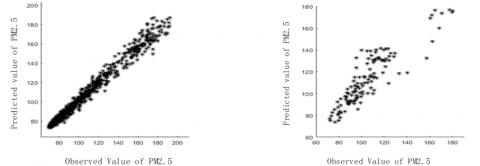a) Global distribution of predicted and observed PM2.5 particle concentration  b) Local distribution of predicted and observed PM2.5 particle concentration

Figure 8. Global and local distribution maps of predicted and observed PM2.5 particle concentration

The experimental results show that, between the predicted value and the observed value generated by the proposed algorithm, there’s a fitting distribution feature that is close to y=X+ε, wherein ε is an arbitrary small integer, representing the similarity between the predicted value and the observed value. The smaller the value of ε, the closer the predicted value to the observed value, then, according to the actual application field of the algorithm, the accuracy requirement and the experimental conclusion, the value of ε was estimated and set. This indicates that the algorithm’s predicted value and observed value were come from the same dataset, therefore, the proposed algorithm has a good prediction effect.

3.7 A comparative study of the prediction performance of the algorithms

Based on the same training and test dataset, different input parameters, and different network structures, the performances of the TS-LSTM algorithm proposed in this paper, the MLR algorithm , the SVR algorithm , the wavelet-ARMA/ARIMA algorithm , the FNN algorithm , the LSTM NN algorithm , the GC-LSTM NN algorithm , and the DL-LSTM NN algorithm  are compared.

The experimental results show that (Table 3), under the condition of same training and test dataset, different input parameters and different network structures, compared with the non-neural network algorithms, the artificial neural network algorithms can extract non-linear features of the evolution process of particle concentration through non-linear mapping methods, and better represent these features, therefore, these algorithms have very good non-linear prediction ability. The deep neural network can effectively extract the deep abstract features of particle concentration and express the essential properties of particle concentration; therefore, the prediction ability of the deep neural network is better than that of the shallow neural network, and it is slightly better than that of the proposed algorithm. The algorithm proposed in this paper combined the LSTM algorithm’s time prediction ability and space prediction ability; it integrated the learning time and the spatial features, its prediction ability outperformed other LSTM networks, and the performance of the algorithm in local area was better than that in the global area. In summary, compared with other time series analysis algorithms, the ST-LSTM algorithm proposed in this paper has better prediction ability.

Table 3. Algorithm performance comparison

 Evaluation Algorithm Global Area Local Area RMSE MAE MAPE RMSE MAE MAPE MLR 17.26 15.01 24.43 15.36 12.09 22.32 SVR 15.28 11.64 17.34 14.22 8.54 14.96 Wavelet-ARMA 16.86 11.07 13.33 15.32 8.21 11.23 Fusing NN 15.59 8.56 12.54 13.09 7.53 10.04 LSTM NN 13.24 7.92 11.54 13.21 7.58 9.96 GC-LSTM 14.81 7.87 10.35 13.28 9.34 10.32 DL-LSTM 6.03 4.56 7.02 6.30 3.89 5.10 The proposed 9.16 5.21 7.12 7.87 5.32 8.81
4. Conclusions

This paper proposed a novel LSTM algorithm based on spatial-temporal correlations and applied it to the simulation and prediction of the evolution process of the concentration of air pollutant particles. The paper aimed to solve the problem that traditional LSTM algorithm hasn’t fused the temporal and spatial correlations of the particle concentration, and it achieved the fusion of the time dependence and spatial correlation of the air pollutant particle concentration based on the LSTM algorithm. In the global (the daily PM2.5 concentration data collected from 30 air quality monitoring stations in Beijing-Tianjin-Hebei region from Jan. 2103 to Dec. 2018) and local (the every hour PM2.5 concentration data collected from 12 air quality monitoring stations in Beijing from Jan. 1st , 2015 to Dec. 30th, 2018) dataset, the proposed algorithm had achieved good simulation and prediction results of the evolution process of the concentration of air pollutant particles. Under the condition of same dataset, different network structures and different experimental parameters, the proposed algorithm was compared with various classic algorithms, and the results showed that the algorithm proposed in this paper had good prediction ability and simulation effects. The research found that, in terms of PM2.5 particle concentration evolution simulation and numerical prediction:

1) The performance of deep neural networks is better than that of shallow neural networks; and the performance of shallow neural networks is better than that of non-neural networks.

2) LSTM neural networks perform well in learning long-term time dependence of air concentration; therefore, this type of algorithm outperforms similar shallow neural networks in simulation and prediction performance.

3) The multi-layer deep LSTM neural network with spatial-temporal correlation learning ability has better performance than traditional time series algorithms and neural network algorithms.

4) The algorithm proposed in this paper has good prediction performance and simulation effects on both global and local air quality prediction.

Acknowledgment

This work is supported by the Social Science Fund of Hebei Province of China (Grant numbers: HB18TJ004).

References

 Zheng, Y., Liu, F., Hsieh, H.P. (2013). U-Air: When urban air quality inference meets big data. In Proceedings of the 19th ACM SIGKDD International Conference on Knowledge Discovery and Data Mining, pp. 1436-1444. https://doi.org/10.1145/2487575.2488188

 Di, Q., Dai, L., Wang, Y., Zanobetti, A., Choirat, C., Schwartz, J.D., Dominici, F. (2017). Association of short-term exposure to air pollution with mortality in older adults. JAMA, 318(24): 2446-2456. https://doi.org/10.1001/jama.2017.17923

 Huang, L., Zhang, C., Bi, J. (2017). Development of land use regression models for PM2.5, SO2, NO2 and O3 in Nanjing, China. Environmental Research, 158: 542-552. https://doi.org/10.1016/j.envres.2017.07.010

 Ge, B., Gm, J. (1976). Time Series Analysis: Forecasting and Control rev. ed. Oakland, California, Holden-Day, 31(4): 238-242.

 Saide, P.E., Carmichael, G.R., Scott, N., Gallardo, L., Osses, A.E., Mena-Carrasco, M.A., Pagowski, M. (2011). Forecasting urban PM10 and PM2.5 pollution episodes in very stable nocturnal conditions and complex terrain using WRF-Chem CO tracer model. Atmospheric Environment, 45(16): 2769-2780. https://doi.org/10.1016/j.atmosenv.2011.02.001

 Kim, Y., Fu, J.S., Miller, T.L. (2010). Improving ozone modeling in complex terrain at a fine grid resolution – Part II: Influence of schemes in MM5 on daily maximum 8-h ozone concentrations and RRFs (Relative Reduction Factors) for SIPs in the non-attainment areas. Atmospheric Environment, 44(17): 2116-2124. https://doi.org/10.1016/j.atmosenv.2010.02.038

 Jeong, J.I., Park, R.J., Woo, J.H., Han, Y.J., Yi, S.M. (2011). Source contributions to carbonaceous aerosol concentrations in Korea. Atmospheric Environment, 45(5): 1116-1125. https://doi.org/10.1016/j.atmosenv.2010.11.031

 Stern, R., Builtjes, P., Schaap, M., Timmermans, R., Vautard, R., Hodzic, A., Wolke, R. (2008). A model inter-comparison study focussing on episodes with elevated PM10 concentrations. Atmospheric Environment, 42(19): 4567-4588. https://doi.org/10.1016/j.atmosenv.2008.01.068

 Zhang, H., Zhang, S., Wang, P., Qin, Y., Wang, H. (2017). Forecasting of particulate matter time series using wavelet analysis and wavelet-ARMA/ARIMA model in Taiyuan, China. Journal of The Air & Waste Management Association, 67(7), 776-788. https://doi.org/10.1080/10962247.2017.1292968

 Yoon, H., Jun, S.C., Hyun, Y., Bae, G.O., Lee, K.K. (2011). A comparative study of artificial neural networks and support vector machines for predicting groundwater levels in a coastal aquifer. Journal of Hydrology, 396(1): 128-138. https://doi.org/10.1016/j.jhydrol.2010.11.002

 Lu, W.Z., Wang, W.J., Fan, H.Y., Leung, A.Y.T., Xu, Z.B., Lo, S.M., Wong, J.C.K. (2002). Prediction of pollutant levels in causeway bay area of Hong Kong using an improved neural network model. Journal of Environmental Engineering, 128(12): 1146-1157. https://doi.org/10.1061/(ASCE)0733-9372(2002)128:12(1146)

 Paschalidou, A.K., Karakitsios, S., Kleanthous, S., Kassomenos, P.A. (2011). Forecasting hourly PM10 concentration in Cyprus through artificial neural networks and multiple regression models: Implications to local environmental management. Environmental Science and Pollution Research, 18(2): 316-327. https://doi.org/10.1007/s11356-010-0375-2

 Ong, B.T., Sugiura, K., Zettsu, K. (2016). Dynamically pre-trained deep recurrent neural networks using environmental monitoring data for predicting PM2.5. Neural Computing and Applications, 27(6): 1553-1566. https://doi.org/10.1007/s00521-015-1955-3

 Prakash, A., Kumar, U., Kumar, K., Jain, V.K. (2011). A wavelet-based neural network model to predict ambient air pollutants’ concentration. Environmental Modeling & Assessment, 16(5): 503-517. https://doi.org/10.1007/s10666-011-9270-6

 Mishra, D., Goyal, P. (2017). Neuro-fuzzy approach to forecast NO2 pollutants addressed to air quality dispersion model over Delhi, India. Aerosol and Air Quality Research, 16(1): 166-174. https://doi.org/10.4209/aaqr.2015.04.0249

 Feng, Y., Zhang, W., Sun, D., Zhang, L. (2011). Ozone concentration forecast method based on genetic algorithm optimized back propagation neural networks and support vector machine data classification. Atmospheric Environment, 45(11): 1979-1985. https://doi.org/10.1016/j.atmosenv.2011.01.022

 Ma, X., Tao, Z., Wang, Y., Yu, H., Wang, Y. (2015). Long short-term memory neural network for traffic speed prediction using remote microwave sensor data. Transportation Research Part C-Emerging Technologies, 54: 187-197. https://doi.org/10.1016/j.trc.2015.03.014

 Li, X., Peng, L., Yao, X., Cui, S., Hu, Y., You, C., & Chi, T. (2017). Long short-term memory neural network for air pollutant concentration predictions: Method development and evaluation. Environmental Pollution, 231(231): 997–1004. https://doi.org/10.1016/j.envpol.2017.08.114

 Bai, Y., Zeng, B., Li, C., Zhang, J. (2019). An ensemble long short-term memory neural network for hourly PM2.5 concentration forecasting. Chemosphere, 222: 286-294. https://doi.org/10.1016/j.chemosphere.2019.01.121

 Qin, D., Yu, J., Zou, G., Yong, R., Zhao, Q., Zhang, B. (2019). A novel combined prediction scheme based on CNN and LSTM for urban PM 2.5 concentration. IEEE Access, 7: 20050-20059. https://doi.org/10.1109/access.2019.2897028

 Zhao, J., Deng, F., Cai, Y., Chen, J. (2019). Long short-term memory - Fully connected (LSTM-FC) neural network for PM2.5 concentration prediction. Chemosphere, 220: 486-492. https://doi.org/10.1016/j.chemosphere.2018.12.128

 Qi, Y., Li, Q., Karimian, H., Liu, D. (2019). A hybrid model for spatiotemporal forecasting of PM2.5 based on graph convolutional neural network and long short-term memory. Science of The Total Environment, 664: 1-10. https://doi.org/10.1016/j.scitotenv.2019.01.333

 Wen, C., Liu, S., Yao, X., Peng, L., Li, X., Hu, Y., Chi, T. (2019). A novel spatiotemporal convolutional long short-term neural network for air pollution prediction. Science of The Total Environment, 654: 1091-1099. https://doi.org/10.1016/j.scitotenv.2018.11.086

 Freeman, B.S., Taylor, G., Gharabaghi, B. (2018). Forecasting air quality time series using deep learning. Journal of The Air & Waste Management Association, 68(8): 866-886. https://doi.org/10.1080/10962247.2018.1459956

 Zhou, Y., Chang, F.J., Chang, L.C., Kao, I.F., Wang, Y.S. (2019). Explore a deep learning multi-output neural network for regional multi-step-ahead air quality forecasts. Journal of Cleaner Production, 209: 134-145. https://doi.org/10.1016/j.jclepro.2018.10.243

 Huang, C.J., Kuo, P.H. (2018). A deep CNN-LSTM model for particulate matter (PM2.5) Forecasting in smart cities. Sensors, 18(7): 2220. https://doi.org/10.3390/s18072220

 Feng, X., Li, Q., Zhu, Y., Hou, J., Jin, L., Wang, J. (2015). Artificial neural networks forecasting of PM2.5 pollution using air mass trajectory based geographic model and wavelet transformation. Atmospheric Environment, 107: 118-128. https://doi.org/10.1016/j.atmosenv.2015.02.030